# spikeinterface peak localization

## peak localization in spikeinterface¶

spikeinterface include several methods for unit or peak localization :

• 'center_of_mass' : classic and fast localization. For instance herdingspikes use this method. It is quite accurate on squared MEA but have string artifact when units are on the border of the probe. So for linear probe this method give poor result on X axis.
• 'monopolar_triangulation' with optimizer='least_square' This method is from Julien Boussard and Erdem Varol from the Paninski lab. This has been presented at NeurIPS see also here
• 'monopolar_triangulation' with optimizer='minimize_with_log_penality' It is an improvement from the same team on the previous method not publish yet.

Here an example how to use.

In :
```%load_ext autoreload
```
In :
```from pathlib import Path

import spikeinterface.full as si

import numpy as np
import matplotlib.pyplot as plt
plt.rcParams["figure.figsize"] = (20, 12)
```
In :
```# local folder
base_folder = Path('/mnt/data/sam/DataSpikeSorting/imposed_motion_nick')

dataset_folder = base_folder / 'dataset1/NP1'
preprocess_folder = base_folder / 'dataset1_NP1_preprocessed'
peak_folder = base_folder / 'dataset1_NP1_peaks'

peak_folder.mkdir(exist_ok=True)
```
In :
```# global kwargs for parallel computing
job_kwargs = dict(
n_jobs=40,
chunk_duration='1s',
progress_bar=True,
)
```
In :
```# read the file
rec
```
Out:
`SpikeGLXRecordingExtractor: 384 channels - 1 segments - 30.0kHz - 1957.191s`
In :
```fig, ax = plt.subplots()
si.plot_probe_map(rec, ax=ax)
ax.set_ylim(-150, 200)
```
Out:
`(-150.0, 200.0)`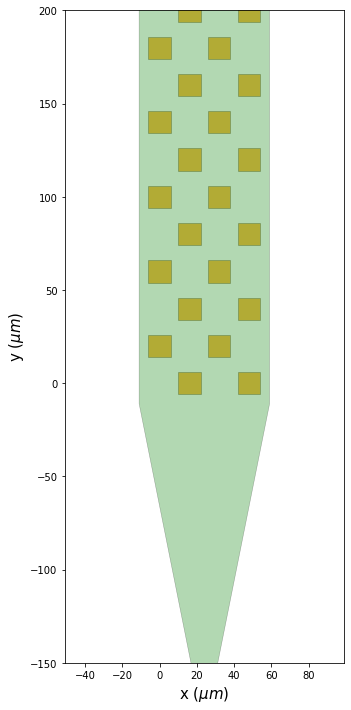### preprocess¶

This take 4 min for 30min of signals

In :
```if not preprocess_folder.exists():
rec_filtered = si.bandpass_filter(rec, freq_min=300., freq_max=6000.)
rec_preprocessed = si.common_reference(rec_filtered, reference='global', operator='median')
rec_preprocessed.save(folder=preprocess_folder, **job_kwargs)
```
```write_binary_recording with n_jobs 40  chunk_size 30000
```
```write_binary_recording: 100%|██████████| 1958/1958 [03:09<00:00, 10.34it/s]
```
In :
```# plot and check spikes
si.plot_timeseries(rec_preprocessed, time_range=(100, 110), channel_ids=rec.channel_ids[50:60])
```
Out:
`<spikeinterface.widgets.timeseries.TimeseriesWidget at 0x7f894ea61af0>`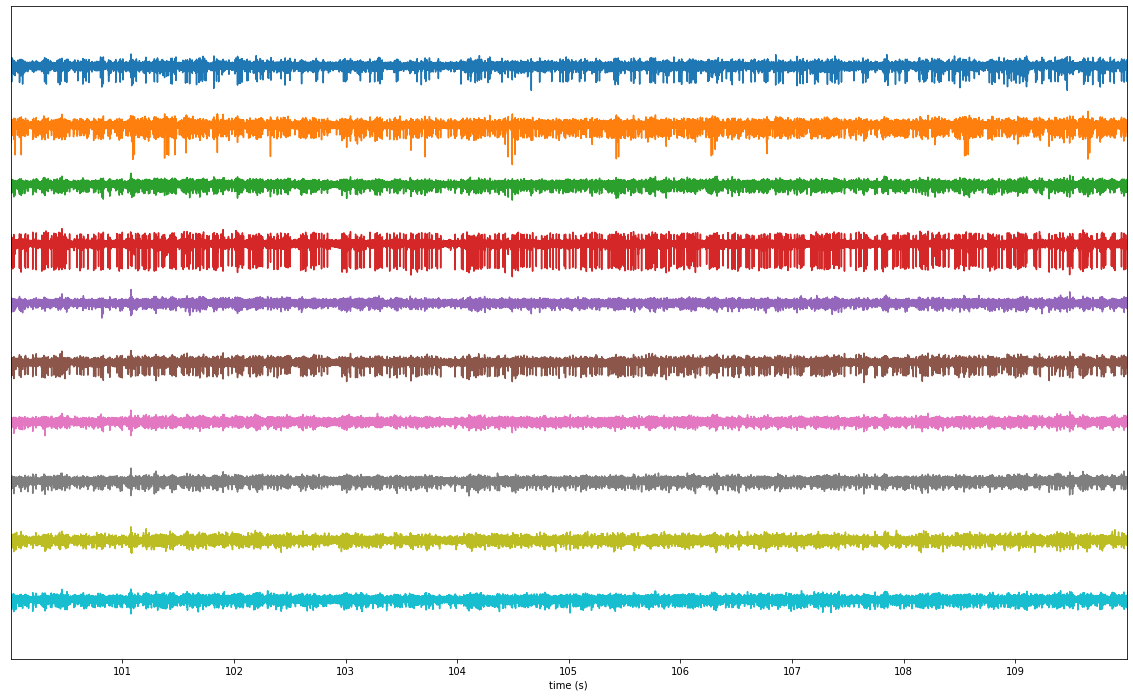### estimate noise¶

In :
```noise_levels = si.get_noise_levels(rec_preprocessed, return_scaled=False)
fig, ax = plt.subplots(figsize=(8,6))
ax.hist(noise_levels, bins=np.arange(0,10, 1))
ax.set_title('noise across channel')
```
Out:
`Text(0.5, 1.0, 'noise across channel')`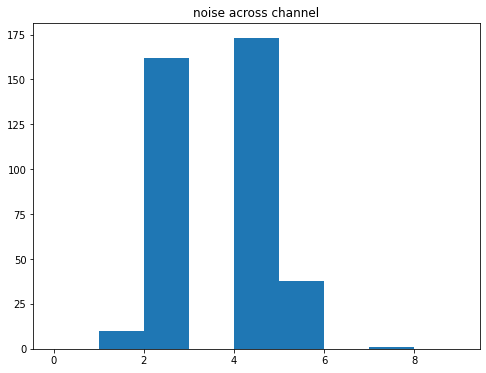### detect peaks¶

This take 1min10s

In :
```from spikeinterface.sortingcomponents.peak_detection import detect_peaks
```
In :
```if not (peak_folder / 'peaks.npy').exists():
peaks = detect_peaks(
rec_preprocessed,
method='locally_exclusive',
peak_sign='neg',
detect_threshold=5,
n_shifts=5,
noise_levels=noise_levels,
**job_kwargs,
)
np.save(peak_folder / 'peaks.npy', peaks)
print(peaks.shape)
```
```detect peaks: 100%|██████████| 1958/1958 [01:11<00:00, 27.26it/s]
```
```(4041179,)
```

### localize peaks¶

We use 2 methods:

• 'center_of_mass': 9 s
• 'monopolar_triangulation' leagacy : 26min
• 'monopolar_triangulation' log barrier : 16min
In :
```from spikeinterface.sortingcomponents.peak_localization import localize_peaks
```
In :
```if not (peak_folder / 'peak_locations_center_of_mass.npy').exists():
peak_locations = localize_peaks(
rec_preprocessed,
peaks,
ms_before=0.3,
ms_after=0.6,
method='center_of_mass',
**job_kwargs,
)
np.save(peak_folder / 'peak_locations_center_of_mass.npy', peak_locations)
print(peak_locations.shape)
```
```localize peaks: 100%|██████████| 1958/1958 [00:08<00:00, 218.72it/s]
```
```(4041179,)
```
In :
```if not (peak_folder / 'peak_locations_monopolar_triangulation_legacy.npy').exists():
peak_locations = localize_peaks(
rec_preprocessed,
peaks,
ms_before=0.3,
ms_after=0.6,
method='monopolar_triangulation',
method_kwargs={'local_radius_um': 100., 'max_distance_um': 1000., 'optimizer': 'least_square'},
**job_kwargs,
)
np.save(peak_folder / 'peak_locations_monopolar_triangulation_legacy.npy', peak_locations)
print(peak_locations.shape)
```
```localize peaks: 100%|██████████| 1958/1958 [26:42<00:00,  1.22it/s]
```
```(4041179,)
```
In :
```if not (peak_folder / 'peak_locations_monopolar_triangulation_log_limit.npy').exists():
peak_locations = localize_peaks(
rec_preprocessed,
peaks,
ms_before=0.3,
ms_after=0.6,
method='monopolar_triangulation',
method_kwargs={'local_radius_um': 100., 'max_distance_um': 1000., 'optimizer': 'minimize_with_log_penality'},
**job_kwargs,
)
np.save(peak_folder / 'peak_locations_monopolar_triangulation_log_limit.npy', peak_locations)
print(peak_locations.shape)
```
```localize peaks: 100%|██████████| 1958/1958 [16:15<00:00,  2.01it/s]
```
```(4041179,)
```
In :
```# load back
# peak_locations = np.load(peak_folder / 'peak_locations_center_of_mass.npy')
# peak_locations = np.load(peak_folder / 'peak_locations_monopolar_triangulation_legacy.npy')
print(peak_locations)
```
```[( 18.81849235, 1782.84538913,  78.17532357, 1696.96239445)
( 31.90279769, 3847.75061369, 134.79844077, 1716.03155721)
(-23.12038001, 2632.87834759,  87.76916268, 2633.62546695) ...
( 40.0839554 , 1977.83852796,  26.50998809, 1092.53885299)
(-51.40036701, 1772.34521905, 170.65660676, 2533.03617278)
( 54.3813594 , 1182.28971165,  87.35020554, 1303.53392431)]
```

### plot on probe¶

In :
```for name in ('center_of_mass', 'monopolar_triangulation_legacy', 'monopolar_triangulation_log_limit'):

probe = rec_preprocessed.get_probe()

fig, axs = plt.subplots(ncols=2, sharey=True, figsize=(15, 10))
ax = axs
plot_probe(probe, ax=ax)
ax.scatter(peak_locations['x'], peak_locations['y'], color='k', s=1, alpha=0.002)
ax.set_xlabel('x')
ax.set_ylabel('y')
ax.set_title(name)
if 'z' in peak_locations.dtype.fields:
ax = axs
ax.scatter(peak_locations['z'], peak_locations['y'], color='k', s=1, alpha=0.002)
ax.set_xlabel('z')
ax.set_ylim(1500, 2500)
```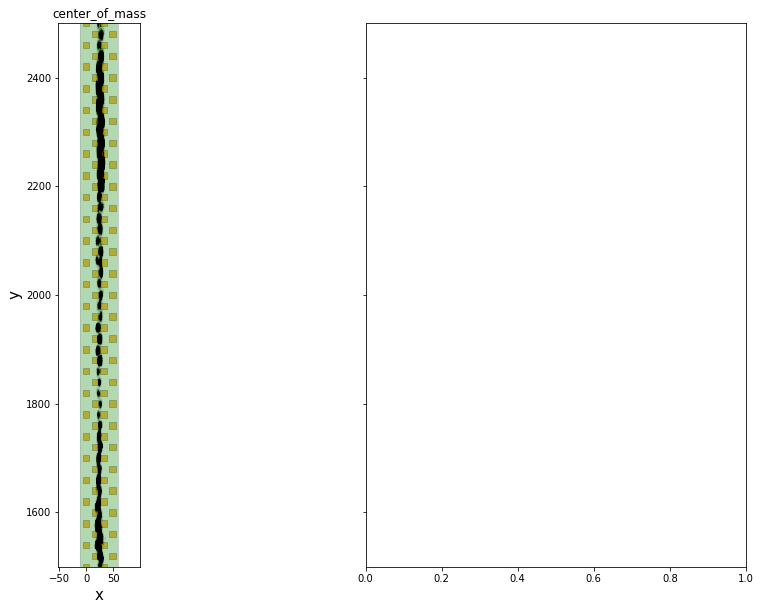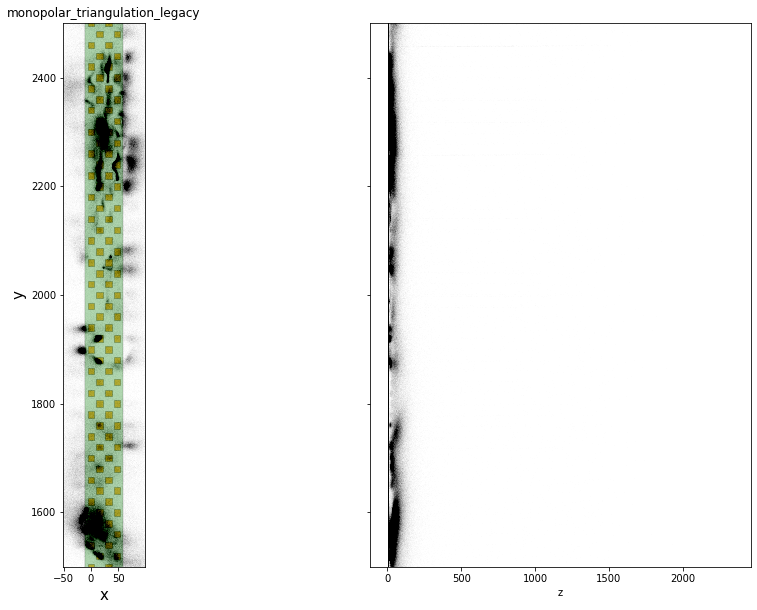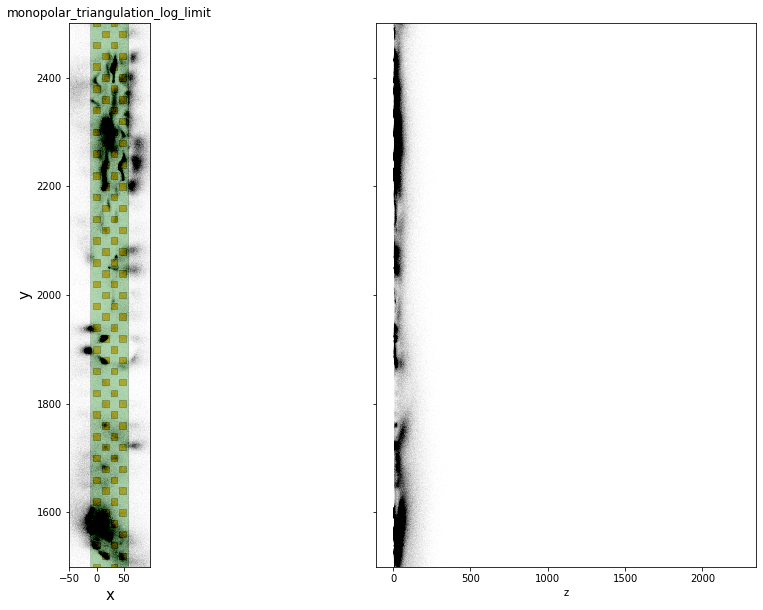### plot peak depth vs time¶

In :
```fig, ax = plt.subplots()
x = peaks['sample_ind'] / rec_preprocessed.get_sampling_frequency()
y = peak_locations['y']
ax.scatter(x, y, s=1, color='k', alpha=0.05)
ax.set_ylim(1300, 2500)
```
Out:
`(1300.0, 2500.0)`